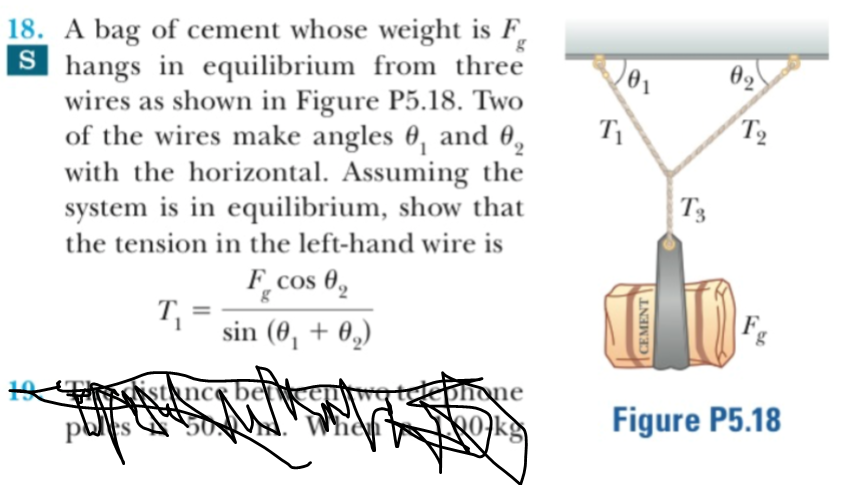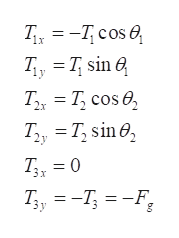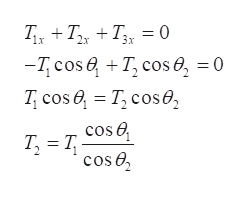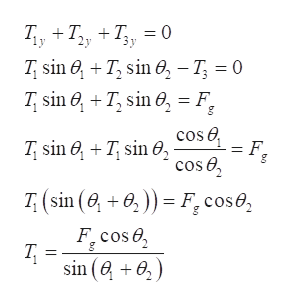# 18. A bag of cement whose weight is F,Shangs in equilibrium from threewires as shown in Figure P5.18. Twoof the wires make angles 0, and 0with the horizontal. Assuming thesystem is in equilibrium, show thatgө2T2T'3the tension in the left-hand wire isF_cosgsin (00,ksqncs bertkaurg teeononeWhenFigure P5.18OkgCEMENT

Question
26 viewshelp_outlineImage Transcriptionclose18. A bag of cement whose weight is F, Shangs in equilibrium from three wires as shown in Figure P5.18. Two of the wires make angles 0, and 0 with the horizontal. Assuming the system is in equilibrium, show that g ө2 T2 T'3 the tension in the left-hand wire is F_cos g sin (00, ksqncs bertkaurg teeonone When Figure P5.18 Okg CEMENT fullscreen
check_circle

Step 1

Write the components of the tension along vertical and horizontal direction. Take upward and right direction as positive and left and downward direction as negative for reference.help_outlineImage Transcriptionclose= -Tcos e 1x = T7 sin Q Tax T cos e Ty T sin T3 0 T,, %3 —Т, %3 —F, y fullscreen
Step 2

Write the expression of force equilibrium at the junction in x direction.help_outlineImage TranscriptioncloseT TT = 0 3x -Tcos T cos e, 0 T cos T, cos cos e Т, %3D Т, cos e fullscreen
Step 3

Write the expression of force equilibriu...help_outlineImage TranscriptioncloseТ. Т, + T,, — 0 T sin T sin , - T 0 T, sin +T, sin F sin +7,sin , cos cos e L = Fe (sin (+)) F cose F.cose sin ( ) fullscreen

### Want to see the full answer?

See Solution

#### Want to see this answer and more?

Solutions are written by subject experts who are available 24/7. Questions are typically answered within 1 hour.*

See Solution
*Response times may vary by subject and question.
Tagged in

### Kinematics